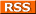Comments Rss
PostGIS ST_GeomFromText

The ST_GeomFromText OGC function is used to convert a Well Known Text (WKT) version of a geometry into a PostGIS geometry. Aliases: ST_GeometryFromText
SQL-MM Spec Equivalents ST_GeomFromText, ST_WKTToSQL ()

## Example inserting a linestring

This example demonstrates using a WKT representation of a Street in NAD 83 LongLat format and inserting it into a PostGIS geometry field.

``` INSERT INTO streets(street_name, the_geom) SELECT 'some street', ST_GeomFromText('LINESTRING(-70.729212 42.373848,-70.67569 42.375098)',4269) ```

The ST_GeomFromText function or equivalent exists in other spatial databases. For example in MySQL 5 and above, the function is called the same and uses the same syntax as above. In Oracle 10g Spatial and Locator, you would use the SDO_GEOMETRY function. So the above in Oracle would look something like. ``` ```

``````INSERT INTO streets(street_name, the_geom)
SELECT 'some street',
SDO_GEOMETRY('LINESTRING(-70.729212 42.373848,-70.67569 42.375098)',SDO_CS.MAP_EPSG_SRID_TO_ORACLE(4269)))
FROM DUAL
``````
``` ``` Note in the above code we are using an Oracle function called SDO_CS.MAP_EPSG_SRID_TO_ORACLE(), because Oracle Spatial Reference System IDs (SRID) are usually not the same as the commonly used European Petroleum Survey Group (EPSG)standard codes. PostGIS and MySQL SRIDs are generally the same as the EPSG IDs so that call is not necessary.

## Example inserting a multipolygon

``` INSERT INTO boston_buildings(name, the_geom) VALUES('some name', ST_GeomFromText('MULTIPOLYGON(((235670.354215375 894016.780856,235668.324215375 894025.050856,235681.154215375 894028.210856,235683.184215375 894019.940856,235670.354215375 894016.780856)))', 2805) ) ```